Next: 2.2 Silicon Up: 2.1 Graphene Previous: 2.1.1 Electronic Structure   Contents

## 2.1.2 Phononic Structure

The phonon bandstructure can be described by first principle models [35,36], the valence force field (VFF) method [37,38], and the force constant method (FCM). The latter has the lowest computation time requirements. In this model, the dynamics of atoms are simply described by a few force springs connecting an atom to its surroundings up to given numbers of neighbors. In contrast, the VFF method is based on the evaluation of the force constants , which requires a much larger computational times. The FCM uses a small set of empirical fitting parameters and can be easily calibrated to experimental measurements. Despite its simplicity, it can provide accurate and transferable results [40,41]. Thus, it is a convenient and robust method to investigate thermal properties of crystals and in particular of graphene nanostructures.

Table 2.1: The fitting parameters of the force constant tensor in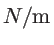for graphene .
N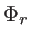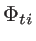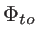1 365.0 245.0 98.2
2 88.0 -32.3 -4.0
3 30.0 -52.5 1.5
4 -19.2 22.9 -5.8

The FCM model we employ involves a fourth nearest-neighbor approximation (see Fig. 2.2). The force constant tensor describing the coupling between the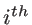and the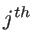carbon atom, which are the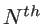nearest-neighbor of each other, is given by: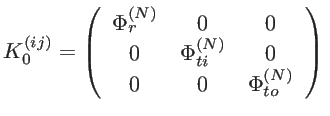(2.7)

where,,andare the radial, the in-plane transverse, and the out-of-plane transverse components of the force constant tensor, respectively. Their values are presented in Table 2.1 .

The motion of the atoms can be described by a dynamic matrix as: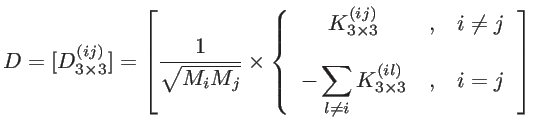(2.8)

where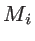is the atomic mass of thecarbon atom, and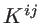is a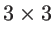force constant tensor describing the coupling between theand thecarbon atom. In Cartesian coordinates it is given by: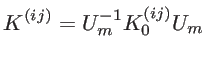(2.9)

where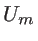is a unitary matrix defined as: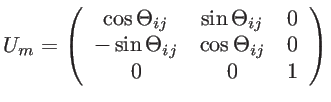(2.10)

Here, we assume that the graphene sheet is located in the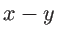plane and that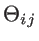represents the angle between the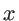-axes and the bond between theandcarbon atom. The phononic bandstructure can be calculated by solving the eigen-value problem described by: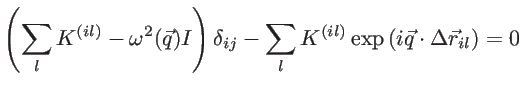(2.11)

where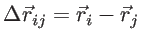is the distance between theand thecarbon atom, and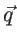is the wave vector. Equivalently, after setting up the dynamic matrix, one can use the following eigen-value problem: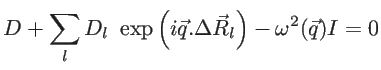(2.12)

where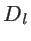is the dynamic matrix representing the interaction between the unit cell and its neighboring unit cells separated by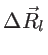.

The phononic bandstructure of graphene shown in Fig. 2.4 is evaluated using the fourth nearest-neighbor FCM with force constants given in Table. 2.1. This method relies on twelve fitting parameters that determine the force constants, which are extracted from experiments. To validate the model, we present the experimental phonon bandstructure results from Refs. [40,43]. As expected, the result is in good agreement with the experimental data (see Fig. 2.4), especially for the low phonon frequencies, which are the most important ones in determining the thermal conductivity.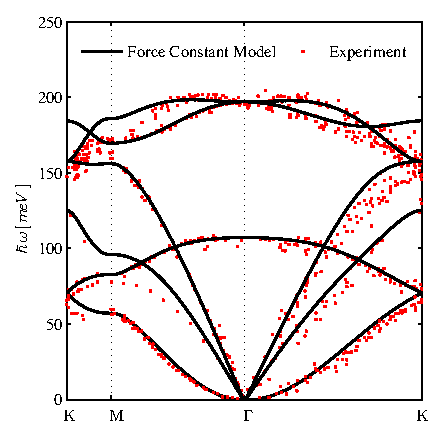Because the model relies on empirical parameters fitted to experiments, it is much more computationally efficient compared to other atomistic formalisms, such as the valence force field (VFF) method. In the VFF method, for example, the force constants for each atom in the unit cell are calculated, and the simulation time is dominated by the dynamic matrix construction . The approximation in that method comes from the parameters used in the evaluation of the potential energy. For FCM, since force constants are empirical parameters, the construction time of the dynamic matrix is negligible, which makes the computation much more efficient. The simulation time is determined by the solution of the eigenvalue problem. In the graphene lattice this results in 18 neighbors for each atom as shown in Fig. 2.2. In FCM we assume that there is a spring between each carbon atom and its 18 neighbors. This number is reduced in the case of boundary atoms with less nearest-neighbors.Next: 2.2 Silicon Up: 2.1 Graphene Previous: 2.1.1 Electronic Structure   Contents
H. Karamitaheri: Thermal and Thermoelectric Properties of Nanostructures